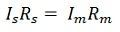# Shunt Resistor

Definition: A resistor having a very low value of resistance such type of resistor is called shunt resistance. The shunt resistor is mainly made of the material having the low-temperature coefficient of resistance. It is connected in parallel with the ammeter whose range is to be extended. It is also connected in series with the load whose current is to be measured.

Consider an ammeter has its resistance Rm and it measured only a small current Im.  A shunt resistor Rs is placed in parallel with the ammeter for extending their range.Let, Rs = Shunt resistance
Is = Shunt current
I = total load or circuit current to be measuredSince shunt is in parallel with ammeter,From above equation we get,Where N is the ratio of the total current (I) to be measured to the full-scale deflection current Im is known as the multiplying power of the shunt.

The shunt is used in the galvanometer for measuring the large current. It is connected in parallel to the circuit of the galvanometer. The galvanometer is the current sensing devices. The direction of flow of current inside the circuit is determined by the pointer of the galvanometer.